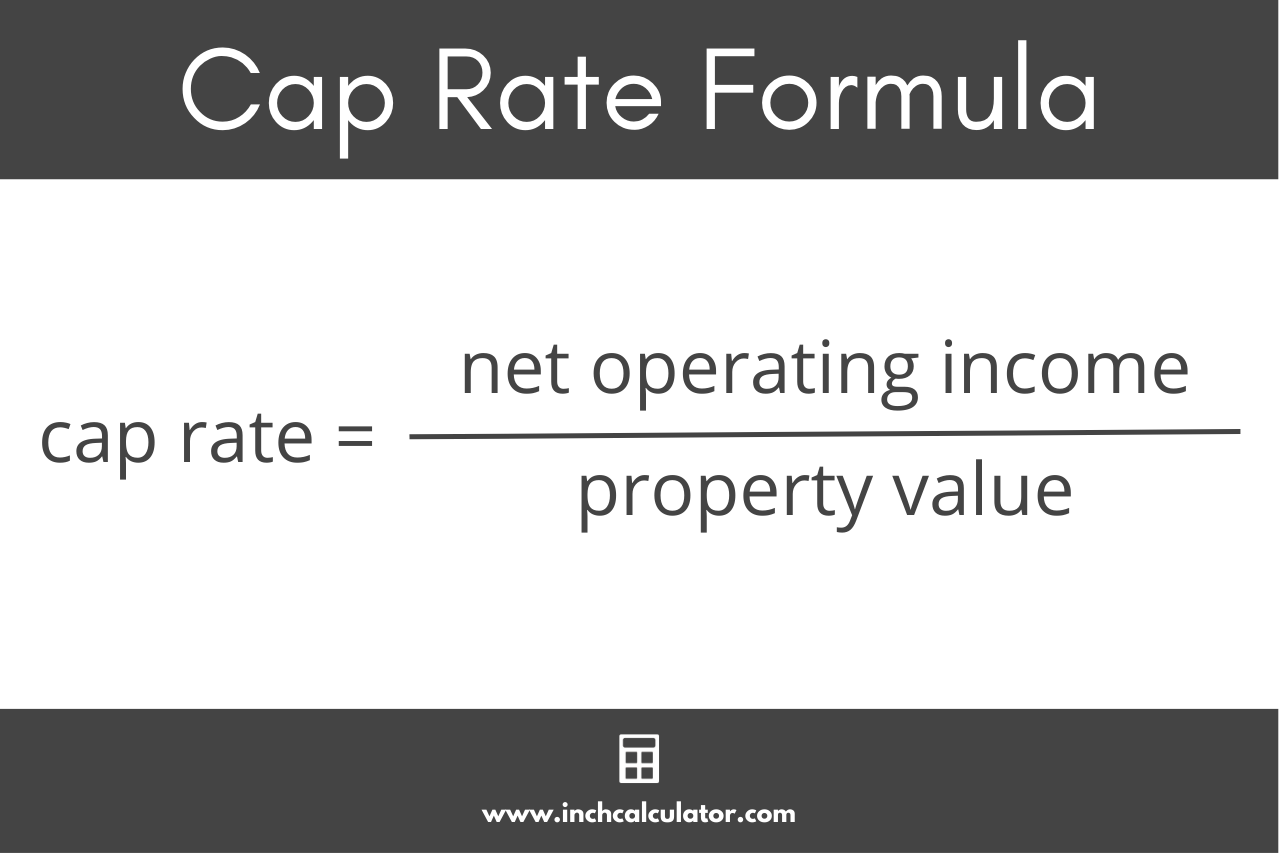# Cap Rate Calculator

Use the cap rate calculator below to find the capitalization rate for a property.

\$
\$
\$

## Cap Rate:

### Net Operating Income

\$
Learn how we calculated this below

## How to Calculate the Cap Rate

When it comes to investing in real estate, one of the most important things to consider is the potential return on investment. One common metric to measure this is the capitalization rate, also called the cap rate.

The cap rate is essentially the rate of return that an investment property generates or is expected to generate. The cap rate is a ratio of the annual net operating income (NOI) for a property relative to its market value, expressed as a percentage.

It’s useful to see how much income a property generates relative to its value, and it’s often used to compare the profitability of different properties.

You can calculate the cap rate by following a few easy steps.

### Step One: Calculate the Net Operating Income

Net operating income (NOI) is the annual revenue generated by an investment property after subtracting operating expenses.

To calculate the NOI, you can subtract the annual operating expenses from the annual income for the property. Operating expenses include expenses such as property taxes, insurance, maintenance costs, and property management fees.

For example, if a property generates \$100,000 in rental income and incurs \$40,000 in expenses, the NOI is \$60,000.

### Step Two: Determine the Property’s Value

The value is the estimated value of the property in the current market. When purchasing an investment property, the purchase price is often used as the value.

You can estimate the current value by analyzing recent sales of similar properties, getting a professional appraisal, or consulting with a real estate agent.

### Step Three: Calculate the Cap Rate

To calculate the cap rate, use the cap rate formula below:

cap rate = net operating income / property value × 100%

So, to find the cap rate, divide the NOI by the market value, then multiply by 100 to get a percentage.For example, let’s find the cap rate for a property with a current value of \$700,000 that has an annual net operating income of \$60,000.

cap rate = \$60,000 / \$700,000 × 100%
cap rate = 0.0857 × 100%
cap rate = 8.57%

So, in this example, the cap rate is 8.57%.

### Considerations When Using Cap Rate

While the cap rate is a useful tool for evaluating the profitability of a rental property, there are some important factors to keep in mind.

First, it’s important to remember that metrics such as the cap rate and cash on cash return only take into account the current income and expenses of a property. They do not account for potential changes in the rental market or future maintenance expenses, such as a major unexpected repair.

For properties that may have income that fluctuates with vacancy or volatile income, a discounted cash flow model is a more accurate way to measure the return.

The cap rate also does not consider the tax advantages, such as rental property depreciation, or the cost of debt in the form of a mortgage.

The primary use of the cap rate is to compare the relative value of similar investment properties, not to accurately estimate the return on investment.

It’s important to remember that the cap rate is just one metric to consider when evaluating a property, and it should always be used in conjunction with other factors, such as location, market trends, and potential expenses.

### Is a lower or higher cap rate better?

In general, a higher cap rate would be considered a better investment because this means the net operating income is higher compared to the value of the investment property.

However, the value of the home, rental market, location of the property, and various other factors also determine whether a real estate investment will be profitable or not and what the typical cap rate should be.

### What is the average cap rate in real estate?

The average and optimal cap rate in real estate is between 5% and 10%. However, cap rate can vary based on the location of an investment property as well as the property type.

A lower cap rate typically denotes lesser risk, but also signals a longer time period for the investor to recoup the original investment.

### Is cap rate the same as ROI?

Cap rate tells you the return on income for a property in relation to its value, typically where the property is purchased in all cash. However, the ROI tells you the return on investment of a property that typically has a mortgage over a period of time.

Both help investors determine if a property is a viable investment, but each tells a slightly different story.